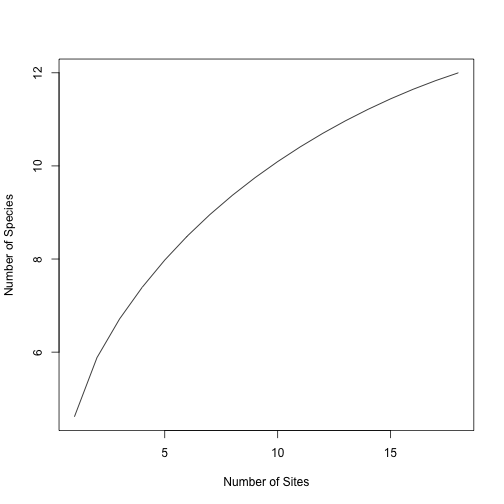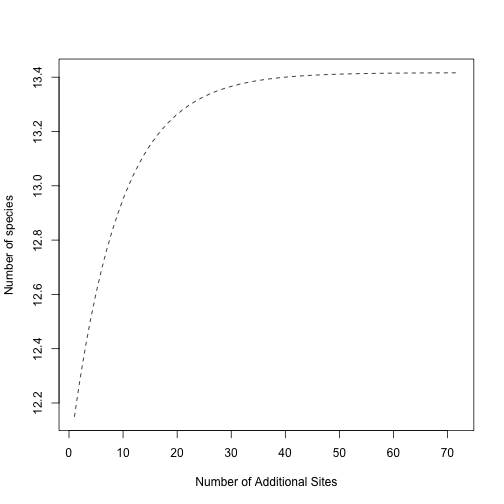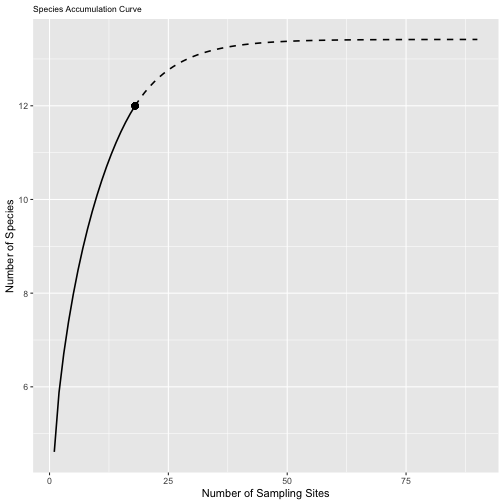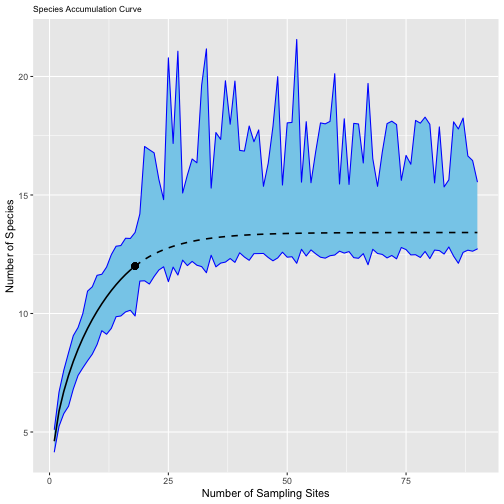Featured

## Coding blog

On this page, I post bits of R code that I’m excited about… mine and other peoples’. As I develop them, I will link to R packages I create.

If you’re interested in what I’m up to, you can peruse my GitHub. I learn a lot from Stack Overflow, and try to give back to that community.

## `annotate` is crisp; `geom_text` is blurry

I just bumped into a little issue in ggplot2 that makes sense, but I was surprised. It looks like there are two things going on, one of which is a very basic thing about how ggplot2 adds `geom`‘s, but the other is about how various plotting devices deal with overlapping data.

As recognized a decade ago, if you use `geom_text` to print a label without specifying a `data.frame`, the `geom` will use the aesthetics passed previously in the `ggplot` call, and can plot the same data many times in the same location. The function `ggplot2::annotate()` deals with this elegantly by defining a mini `geom` that doesn’t map data from a `data.frame` at all, but instead passes simple vectors defined in the `annotate` call. I just added a little answer (copied here) to a decade-old question on Stack Overflow, illustrating this difference.

The thing I still don’t entirely understand is why plotting the same text over and over in the same place creates the fuzz, but I’ll save that rabbit hole for another day.

``````# load library
library(ggplot2)

# example plot
df <- data.frame("x" = c(1,2,3,4),"y" = c(15,19,35,47))

p <- ggplot(df, aes(x,y)) +
geom_point(size=1) +
geom_smooth(method = "lm", se=FALSE, formula=y~x)

# lousy resolution from `geom_text()`

p + geom_text(aes(x = 3.5, y = 37, label ="123456789"),size=12, parse = TRUE)

# but fine resolution from `annotate()`

p + annotate("text", x = 3.5, y = 37, label ="123456789", size = 12)``````

# Background

This is a guest post written by Joseph Mack

This post, written by Joseph Mack, explores the working of the unified
interpolation/extrapolation tools described in Colwell et al. 2012 Journal of
Plant Ecology
“Models and estimators linking individual-based and sample-based
rarefaction, extrapolation and comparison of assemblages” doi:
10.1093/jpe/rtr044. Joseph shared this tutorial with the Fall 2021 Biodiversity
Measurement seminar.

# Joseph’s tutorial

First, we must import a sample presence-absence dataset.

``````spp_incidence <- read.csv("data/ann_incidence.csv", row.names = 1)
``````

The equations used in this analysis are derived from a Bernoulli Product model.
Under this model, species \(i\) has probability theta of occurring. What is the
probability that species \(i\) is not present in a given site?

Do we know this probability for each species in our dataset?

To proceed, we must determine how many species (`Sobs`) and sites (`sites`) are
in the data set (`Sobs`)?

``````sites <- length(spp_incidence)
Sobs <- nrow(spp_incidence)
sites
``````
```##  18
```
``````Sobs
``````
```##  12
```

The equation below can be found on pg. 6 of Colwell et al. 2012. It calculates
the incidence-based frequency of a given species.

``````#What is the incidence-based frequency (Yi) of Sp1?
Ysp1 = sum(as.numeric(spp_incidence["sp1",]))
Ysp1
``````
```##  NA
```
``````#What about the other species?
spp_names = rownames(spp_incidence)
Yi <- c(1:12)
for (i in 1:12) {
Yi[i] <- sum(spp_incidence[i,])
}
``````

The code below creates a table of incidence frequencies (the row sums of our
data set), which will be used to calculate `Qk`.

``````incidence_freqs <- data.frame(cbind(Yi, spp_names))
``````

Next, we must determine how many ‘unique’ species are in the dataset. In other
words, how many species occur in only one site? This requires us to calculate
`Qk`, the incidence frequency count and an essential statistic for sample-based
extrapolation.

``````Q <- function(k) {
out = 0
for (i in 1:length(incidence_freqs\$Yi)) {
if (incidence_freqs\$Yi[i] == k) {
out = out + 1
}
}
return(out)
}

#How many species are found in only 1 site? 4 sites? 9 of the sites? All the sites?
Q(1)
``````
```##  3
```
``````Q(4)
``````
```##  1
```
``````Q(9)
``````
```##  0
```
``````Q(18)
``````
```##  1
```

Below is a function to interpolate/rarefy the sample dataset (equation 17 in
Colewll et al.). It computes the number of species we would recover if we
sampled from `x` fewer sites (`x < sites`).

``````Ssample_rare <- function(x) {
for (i in 1:Sobs) {
out <- (choose((sites - as.numeric(incidence_freqs\$Yi[i])), x))/
choose(sites, x)
}
return(Sobs - sum(out))
}

#How many species would we recover if we sampled from only 1 site? 5 sites? 9 sites?
Ssample_rare(1)
``````
```##  11.05556
```
``````Ssample_rare(5)
``````
```##  11.27778
```
``````Ssample_rare(9)
``````
```##  11.5
```

The values for `Ssample_rare` don’t look right. This rarefaction function is
broken! Can you figure out why? Hint: what is `[i]` doing in the code below?

The rarefaction code below works! Why?

``````Ssample_rare <- function(x) {
out = c()
for (i in 1:Sobs) {
out[i] <- (choose((sites - as.numeric(incidence_freqs\$Yi[i])), x))/choose(sites, x)
}
return(Sobs - sum(out))
}

#NOW, how many species would we recover if we sampled from only 1 site? 5 sites? 9 sites?
Ssample_rare(1)
``````
```##  4.611111
```
``````Ssample_rare(5)
``````
```##  7.977708
```
``````Ssample_rare(9)
``````
```##  9.748416
```
``````#These values make more sense!
``````

Now that we have a means to interpolate our data, we can make a rarefaction plot.

``````diversity_r = sapply(1:18, Ssample_rare) # This outputs a vector of Ssample_rare
# outputs for 1 to 18 sampling sites.
plot(1:18
, diversity_r
, type = "l"
, ylab = "Number of Species"
, xlab = "Number of Sites")
``````Next, we can work on extrapolating the dataset `(Ssample(sites + s))` using
equations 18 and 21 from Colwell et al. 2012. This addresses how many species we
would find if we sampled `s` additional sites.

To do this, we first need to estimate `Q(0)`: the number of species absent from
our samples.

``````#Because Q(2) > 0 for our data set, we can use the following equation based on the Chao2 estimator:
Q0 = ((sites-1)/sites)*((Q(1)^2)/(2*Q(2)))
Q0
``````
```##  1.416667
```

With a value for `Q0`, we can implement the authors’ equation for extrapolation
as a function of `s` additional samples:

``````Ssample_extr <- function(s) {
out <- Sobs + (Q0*(1 - (1 - (Q(1)/(Q(1) + (sites*Q0))))^s))
return(out)
}

#How many species would we recover if we sampled 2 extra sites? 50 extra sites?
Ssample_extr(2)
``````
```##  12.28255
```
``````Ssample_extr(50)
``````
```##  13.41122
```
``````#Make an extrapolation plot for 72 additional sites.
diversity_e = sapply(1:72, Ssample_extr) #This outputs a vector of Ssample_extr
# outputs for 1 to 50 additional sampling sites.
plot(1:72
, diversity_e
, type = "l"
, lty = 2
, ylab = "Number of species"
, xlab = "Number of Additional Sites")
``````Now, we can combine the rarefaction and extrapolation plots into a single plot:

``````library(ggplot2)
combined_diversity = c(diversity_r, diversity_e) #Combining the vectors of
# rarefied and extrapolated species counts, for 1 - 90 sites.
combined_diversity
``````
```##    4.611111  5.882353  6.713235  7.391830  7.977708  8.494344  8.955505  9.371041  9.748416 10.093263 10.409754 10.700927
##  10.968954 11.215359 11.441176 11.647059 11.833333 12.000000 12.149123 12.282548 12.401929 12.508744 12.604315 12.689825
##  12.766335 12.834791 12.896041 12.950844 12.999878 13.043750 13.083005 13.118127 13.149552 13.177669 13.202827 13.225337
##  13.245477 13.263497 13.279620 13.294046 13.306953 13.318502 13.328835 13.338081 13.346353 13.353754 13.360377 13.366302
##  13.371603 13.376347 13.380591 13.384389 13.387786 13.390826 13.393546 13.395980 13.398158 13.400106 13.401849 13.403409
##  13.404804 13.406053 13.407170 13.408170 13.409064 13.409865 13.410581 13.411221 13.411794 13.412307 13.412766 13.413177
##  13.413544 13.413873 13.414167 13.414430 13.414665 13.414876 13.415065 13.415233 13.415384 13.415519 13.415640 13.415748
##  13.415845 13.415931 13.416009 13.416078 13.416140 13.416195
```
``````sampling_sites = 1:90 #vector for 18 rarefied sites + 72 additional extrapolated
# sites
sampling_sites
``````
```##    1  2  3  4  5  6  7  8  9 10 11 12 13 14 15 16 17 18 19 20 21 22 23 24 25 26 27 28 29 30 31 32 33 34 35 36 37 38 39 40 41 42
##  43 44 45 46 47 48 49 50 51 52 53 54 55 56 57 58 59 60 61 62 63 64 65 66 67 68 69 70 71 72 73 74 75 76 77 78 79 80 81 82 83 84
##  85 86 87 88 89 90
```
``````diversity_est <-
data.frame(cbind(combined_diversity, sampling_sites))
sac_plot = ggplot(
data = subset(diversity_est, sampling_sites <= 18),
aes(x = sampling_sites, y = combined_diversity)
) +
geom_line(size = 0.75) +
geom_line(
data = subset(diversity_est, sampling_sites >= 18),
aes(x = sampling_sites, y = combined_diversity),
lty = 2,
size = 0.75
) +
geom_point(aes(x = 18, y = 12), size = 3, pch = 16) +
xlab("Number of Sampling Sites") +
ylab("Number of Species") +
ggtitle("Species Accumulation Curve") +
theme(plot.title = element_text(size = 8.5))
``````
``````sac_plot
``````What does the point represent in this plot? The dotted line? The solid line? Is
our data set a good approximation for the total number of species in the region
sampled?

It is possible to obtain a 95% confidence interval about the species
accumulation curve. However, the variance estimators provided by Colwell et al.
2012 are difficult to understand. As an alternative, we can employ
non-parametric bootstrapping to get 95% confidence intervals about the curve.
The code below is not explicitly described in Colwell et al. 2012, but it
employs the same estimators we used above.

Below is a function for getting bootstrap replicates of our rarefaction and
extrapolation estimates.

``````Ssample_boot <- function(x, s) {
# First, we need to build matrices and vectors to hold our boot-strapped data replicates.
boot_data <- matrix(nrow = 12, ncol = 18)
Yi_boot <- c(1:12)
for (i in 1:12) {
# The line below creates a replicate dataset from our original spp_incidence dataset,
# with replacement.
boot_data[i, ] <- rbind(as.numeric(sample(spp_incidence[i, ]
, size = 18
, replace = TRUE)))
#The for loop below calculates the incidence frequencies from the replicated dataset.
for (j in 1:12) {
Yi_boot[j] <- sum(boot_data[j,])
}
}
# Below is the equation for rarefaction that we implemented earlier, where x is
# a sample smaller than sites.
out_rare = c()
for (i in 1:Sobs) {
out_rare[i] <- choose(sites - Yi_boot[i], x)/choose(sites, x)
}

#This calculates a value of Q2 for the replicate data set.
Q2 = sum(Yi_boot == 2)

#This calculates a value of Q1 for replicate data set.
Q1 = sum(Yi_boot == 1)

# We are using a different equation for Q0 here (Equation 22),
# to avoid dividing by 0 when Q2 is 0 in some of our replicates.
Q0_b = ((sites - 1)/sites) * (Q1 * ((Q1 - 1))/(2 * (Q2 + 1)))

# Below is just equation 18, with some special conditions to account for
# scenarios where the function divides by 0.
out_extr <- Q0_b * (1 - (exp((-s * Q1))/(Q1 + sites * (Q0_b))))
if (is.nan(out_extr) == TRUE) {
return(c(Sobs - sum(out_rare), Sobs))
} else {
return(c(Sobs - sum(out_rare), Sobs + out_extr))
}
}
``````

The code below tests that the function is working. The first variable (`x`)
specifies the sample size to be interpolated/rarefied, while the second variable
(`s`) specifies the additional sites to be extrapolated. The output should be
different each time you run it.

``````Ssample_boot(1,2)
``````
```##   4.388889 12.000000
```

The code below gets bootstrap replicates using `replicate` and `mapply`. How
many bootstrap replicates are we running?

``````var1 <- 1:18
var2 <- 1:90
boot_data <- replicate(100, mapply(Ssample_boot, var1, var2))
``````

Now that we have our bootstrap replicates, we can set up an empty matrix to
store our bootstrap replicate data. Each column is a site (1 – 18 interpolated,
19 – 90 extrapolated), and each row is a bootstrapped replicate.

``````combined_boot_data <- matrix(ncol = 90, nrow = 100)

#The code below populates the matrix.
for (i in 1:100) {
combined_boot_data[i, 1:18] <- boot_data[, , i][1, 1:18]
}
for (i in 1:100) {
combined_boot_data[i, 19:90] <- boot_data[, , i][2, 19:90]
}
combined_boot_data <- data.frame(combined_boot_data)
``````

Now that we have bootstrapped replicates for rarefaction and extrapolation, we
can estimate mean, 2.5% , and 97.5% quantiles for each site (1 – 18 rarefied, 19

• 90 extrapolated). These values can then be used to estimate a 95% confidence
interval centered about our sample data.
``````#This creates an empty vector to store the means of our bootstrapped data.
boot_means <- c()

#This estimates the average across 100 bootstrap replicates for each site (1 - 90).
for (i in 1:90) {
boot_means[i] = (sum(combined_boot_data[,i]))/100
}

#This creates an empty data frame to store the 2.5% and 97.5% quantiles of our bootstrapped data.
boot_quantiles <- matrix(nrow = 2, ncol = 90)
boot_quantiles <- data.frame(boot_quantiles)

# This computes the 2.5% and 97.5% quantiles of our data set,
# populating the data frame we created above.
for (i in 1:90) {
boot_quantiles[,i] <- unname(quantile(combined_boot_data[,i], probs = c(0.025, 0.975)))
}
CI_data <- rbind(combined_boot_data, boot_means, boot_quantiles)
``````

With the average, 2.5%, and 97.5% quantiles, we can compute the lower and upper
confidence bounds for each rarefied and extrapolated site.

For each site, the lower confidence bound = sample data – (bootstrap average –
2.5% bootstrap quantile).

``````LCB <- c()
for (i in 1:90) {
LCB[i] <- combined_diversity[i] - (CI_data[101,i] - CI_data[102,i])
}
``````

For each site, the upper confidence bound = sample data + (97.5% bootstrap
quantile – bootstrap average).

``````UCB <- c()
for (i in 1:90) {
UCB[i] <- combined_diversity[i] + (CI_data[103,i] - CI_data[101,i])
}
confidence_intervals <- data.frame(cbind(LCB,UCB))
``````

With this, we can plot 95% confidence intervals about the species accumulation
curve using the LCB and UCB values calculated above. The block of code below is
mostly identical to the plotting section above. What did we change?

``````sampling_sites = 1:90 #18 sites + 72 additional sites
diversity_est <-
data.frame(cbind(combined_diversity, sampling_sites))
sac_plot = ggplot(
data = subset(diversity_est, sampling_sites <= 18),
aes(x = sampling_sites, y = combined_diversity)
) +
geom_ribbon(
confidence_intervals,
mapping = aes(ymin = LCB, ymax = UCB),
fill = "skyblue",
col = "blue"
) + #Use geom_ribbon to plot our upper and lower confidence intervals.
geom_line(size = 0.75) +
geom_line(
data = subset(diversity_est, sampling_sites >= 18),
aes(x = sampling_sites, y = combined_diversity),
lty = 2,
size = 0.75
) +
geom_point(aes(x = 18, y = 12), size = 3, pch = 16) +
xlab("Number of Sampling Sites") +
ylab("Number of Species") +
ggtitle("Species Accumulation Curve") +
theme(plot.title = element_text(size = 8.5))
sac_plot
``````## Scale-dependent biodiversity patterns

Guest Post from Connor Whitaker

I’m a student in a graduate-level discussion-based seminar that Michael is teaching: “Frontiers in Biodiversity Measurement.” As part of the course, we are expected to “take point” on one paper during the semester, lead the discussion, and craft a tutorial in R. We were given a lot of creative liberty with how to structure the tutorial, with the guidance that our classmates would get the most from it if we related the tutorial to the paper we were discussing that week.

Michael sent out an email about a week before the class began asking everyone to fill out a survey to assess knowledge, and also asked one brave (or in my case, impulsive and a little foolhardy) student to lead the discussion for the second week of class. I decided to take him up on the challenge to go first, and ended up with a finished product I’m quite proud of.

The paper for my discussion was the 2013 paper “Scale-dependent effect sizes of ecological drivers on biodiversity: why standardised sampling is not enough” by Jonathan Chase and Tiffany Knight. In a nutshell, the paper goes as follows:

1. The effects of ecological drivers (any phenomenon that causes ecological change) are highly dependent upon scale, but this is not accounted for in many experimental studies.
2. The authors used a model to investigate and compare several biodiversity metrics. Each of these metrics responds differently to variation in biodiversity across scale.
3. They proposed a specific metric, ENSPIE, (the Effective Number of Species calculated from Hurlbert’s (1971) Probability of Interspecific Encounter) to assess to what extent the effects of various ecological drivers are scale-dependent or independent, and demonstrated this through simulating communities and comparing treatments across different metrics.
4. The authors concluded with how researchers could use multiple biodiversity metrics in combination to draw more accurate conclusions and predictions about the direction and magnitude of ecological drivers.

I’ve been interested in modeling community behavior and dynamics for some time. Building off my own curiosity and research interests, I decided to base my tutorial off the paper’s simulation. Along the way, I discovered that one of the authors, Jonathan Chase, contributed to an R package, “mobsim,” which ported much of the functionality of their original simulation (written in Matlab) into R.  This made writing the code in line with my prior level of programming experience.

The tutorial was designed to demonstrate that ENSPIE (Hill-Simpson diversity) is a powerful metric for deducing whether a driver is effecting a scale-dependent change, or if instead, the driver produces consistent effects across scale. This is critical for conservationists on a changing planet. Having a way to detect consistent effects across scale could enable better predictions based on field research, which is often done, by necessity, at a smaller scale than the effects it may be attempting to document. This will enable better understanding broad patterns of various drivers, and when we can and cannot extrapolate results from a smaller scale.

The tutorial code is available on my github. I hope you enjoy playing around with it as much as I enjoyed writing it.

## Seesaw plots illustrate “mean rarity”

When I took a course on group theory, my clever classmates would read the textbook, and a new abstract concept was theirs… they could fill in the examples of the groups themselves, and come up with new examples. I usually couldn’t do this. In order to generalize, first I had to work through all the examples in the textbook. Even then, the abstraction wasn’t guaranteed.

With diversity indices, I wrestle in a similar way. Learning that the control parameter in Hill Diversities “weights species abundances” differently, I had to draw a picture to see it. And I didn’t. But eventually I saw how the control parameter scales species rarities. In the MeanRarity R package we wrote some code to illustrate this concept, and invite you to play with our interactive tool yourself.